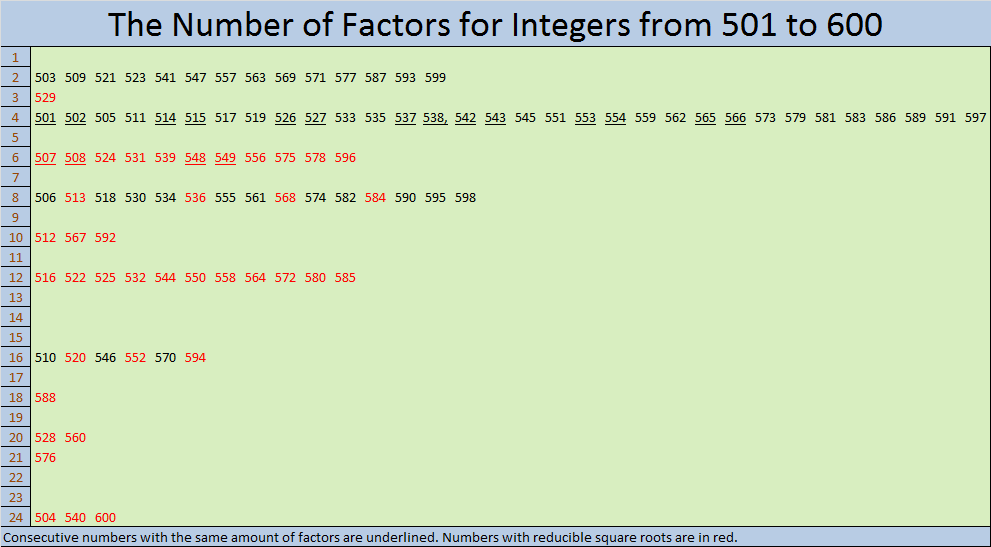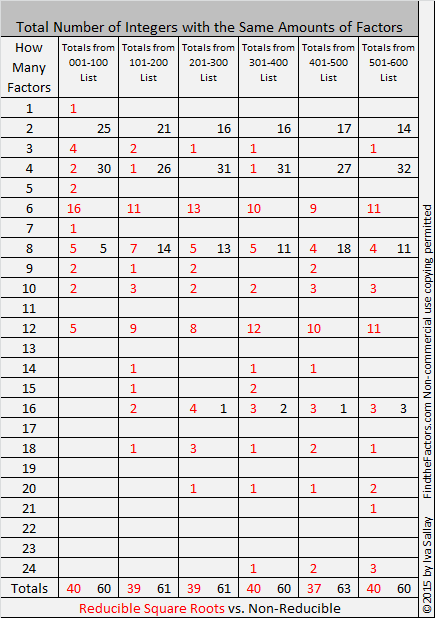# How Many Factors Do the Numbers Up to 600 Have?

600 ties 360, 420, 480, 504, and 540 for having more factors than all other previous whole numbers.

600 is the sum of consecutive prime numbers 293 and 307.

600 is the hypotenuse of two Pythagorean triples: 360-480-600 and 168-576-600. What are the greatest common factors of each of those triples?

In my 300th, 400th, or 500th posts, I reviewed the amount of factors numbers up to 500 have. I even had a horse race for the numbers from 401 to 500 because there were three lead changes which made watching a gif of it a little more interesting.

For the numbers from 501 to 600, there is no horse race: 4 was the first out of the gate and soon left all the other factor amounts in the dust. Click on the graphic below to see it better.I also like to keep track of how many integers have reducible square roots. 40% of these numbers do, but the total numbers from 1 to 600 that have reducible square roots is a little lower than that as you can see in the chart below that also shows the amount of numbers up to 600 which have a particular number of factors:—————————————————————————–

• 600 is a composite number.
• Prime factorization: 600 = 2 x 2 x 2 x 3 x 5 x 5, which can be written 600 = (2^3) x 3 x (5^2)
• The exponents in the prime factorization are 3, 1 and 2. Adding one to each and multiplying we get (3 + 1)(1 + 1)(2 + 1) = 4 x 2 x 3 = 24. Therefore 600 has exactly 24 factors.
• Factors of 600: 1, 2, 3, 4, 5, 6, 8, 10, 12, 15, 20, 24, 25, 30, 40, 50, 60, 75, 100, 120, 150, 200, 300, 600
• Factor pairs: 600 = 1 x 600, 2 x 300, 3 x 200, 4 x 150, 5 x 120, 6 x 100, 8 x 75, 10 x 60, 12 x 50, 15 x 40, 20 x 30 or 24 x 25
• Taking the factor pair with the largest square number factor, we get √600 = (√100)(√6) = 10√6 ≈ 24.494897. (Note: 2 + 4 = 6 and 49 + 48 = 97 so this square root and √6 can be easy to remember.)